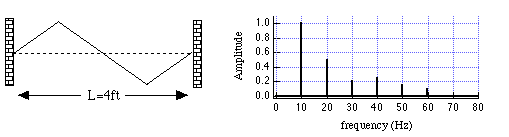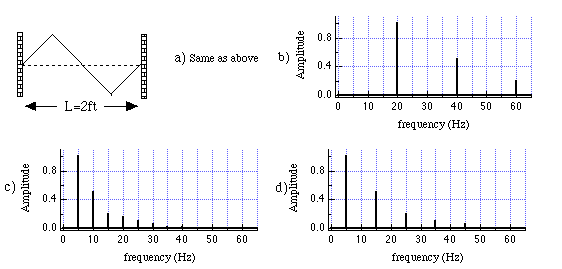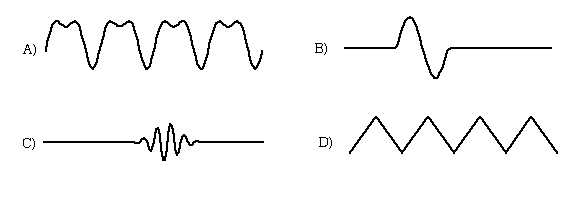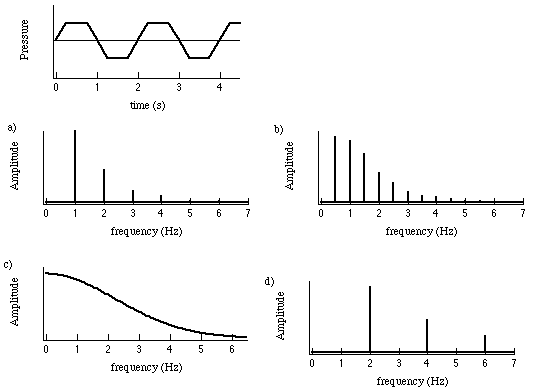TUTORIAL QUESTIONS 300-330

Back to Tutorial Listing

300.  A string of length L that is tied at both ends has allowed frequencies equal to 10 Hz, 20 Hz, 30 Hz, etc.  What would be the fundamental frequency for an identical string that has length L/2?

Fundamentals of Sound reference:Secs. 2-C, 7-F.

310.  A complex standing wave on a string tied at both ends, and its corresponding frequency spectrum, are shown below.Which frequency spectrum would correspond to the complex wave shown below. (Both strings are at the same tension and have the same mass density: i.e., velocity v is the same in both).a)    b)    c)    d)

Fundamentals of Sound reference:Sec. 7-F.

320.  Consider the following traveling pressure sound waves in air:To construct the waves above by Fourier's Theorem (i.e., by adding together sinusoidal waves), which would require a continuous frequency spectrum?

a)  A) and D)
b) A, C), and D)
c) B)
d) B) and C)
e) All of them

Fundamentals of Sound reference:Sec. 7-F.

330.  Which frequency spectrum would most likely correspond to the following sound pressure traveling wave?a)     b)    c)    d)

Fundamentals of Sound reference:Sec. 7-F.

Back to Tutorial Listing